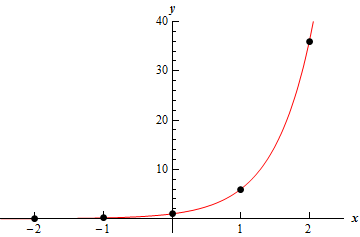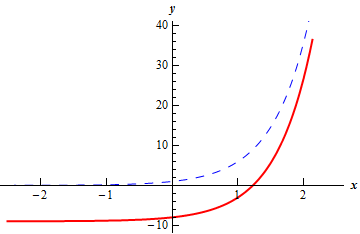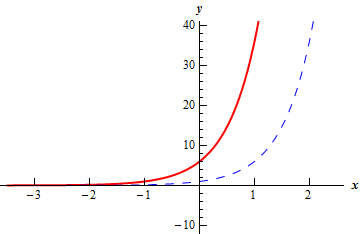Paul's Online Notes
Home / Algebra / Exponential and Logarithm Functions / Exponential Functions
Show Mobile Notice Show All Notes Hide All Notes
Mobile Notice
You appear to be on a device with a "narrow" screen width (i.e. you are probably on a mobile phone). Due to the nature of the mathematics on this site it is best views in landscape mode. If your device is not in landscape mode many of the equations will run off the side of your device (should be able to scroll to see them) and some of the menu items will be cut off due to the narrow screen width.

### Section 6.1 : Exponential Functions

3. Sketch each of the following.

1. $$f\left( x \right) = {6^x}$$
2. $$g\left( x \right) = {6^x} - 9$$
3. $$g\left( x \right) = {6^{x + 1}}$$

Show All Solutions Hide All Solutions

a Show Solution

We can build up a quick table of values that we can plot for the graph of this function.

$$x$$ $$f\left( x \right)$$
-2 $$f\left( { - 2} \right) = {6^{ - 2}} = \frac{1}{{{6^{\,2}}}} = \frac{1}{{36}}$$
-1 $$f\left( { - 1} \right) = {6^{ - 1}} = \frac{1}{6}$$
0 $$f\left( 0 \right) = {6^0} = 1$$
1 $$f\left( 1 \right) = {6^1} = 6$$
2 $$f\left( 2 \right) = {6^2} = 36$$

Here is a quick sketch of the graph of the function.b Show Solution

For this part all we need to do is recall the Transformations section from a couple of chapters ago. Using the “base” function of $$f\left( x \right) = {6^x}$$ the function for this part can be written as,

$g\left( x \right) = {6^x} - 9 = f\left( x \right) - 9$

Therefore, the graph for this part is just the graph of $$f\left( x \right)$$ shifted down by 9.

The graph of this function is shown below. The blue dashed line is the “base” function, $$f\left( x \right)$$, and the red solid line is the graph for this part, $$g\left( x \right)$$.c Show Solution

For this part all we need to do is recall the Transformations section from a couple of chapters ago. Using the “base” function of $$f\left( x \right) = {6^x}$$ the function for this part can be written as,

$g\left( x \right) = {6^{x + 1}} = f\left( {x + 1} \right)$

Therefore, the graph for this part is just the graph of $$f\left( x \right)$$ shifted left by 1.

The graph of this function is shown below. The blue dashed line is the “base” function, $$f\left( x \right)$$, and the red solid line is the graph for this part, $$g\left( x \right)$$.